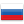### oli30twink's blog

By oli30twink, history, 2 months ago,Hello, codeforces! I am oli30twink

==================

Today I would like to describe this probem: 1878A - How Much Does Daytona Cost? From: Codeforces Round 900 (Div. 3)

I use python to solve it.

Let's start! 1. Number of tests: we should input a nuber and this number would be used in while cycle, so our beginning would be like this:

Solution:

###

2. We should break into two pieces n and k given in one line, so let's write the idea down:

Solution:

###

3. Let's probably do the same with line given us to check(a), it would be like:

Solution:

###

4. Now let's create some variables, we would need:

Solution:

###

5. Use cycle for to compare the number k and all elements from a:

Solution:

###

6. Lastly, we would make the result of their comparage and use s to exclude options, where we print YES NO:

Solution:

Let's sum it all up! Our programm would look like this:

Sum Solution:

Thanks for your attention and good luck!!!Comments (0)